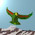## Monday, December 20, 2010

### Dark Matter Update II

In summary, Louise Riofrio's density fractions for baryonic, dark matter and the remainder are given by

$\Omega_b = 1 - \frac{3}{\pi}$, $\Omega_d = \frac{3}{4 \pi}$, $\Omega_r = 3 \Omega_d = \frac{9}{4 \pi}$

and the total density is $\Omega = 1$. The number $\Omega_d = 3/(4 \pi)$ characterises all three densities, showing that Riofrio's law of pair creation and annihilation governs the mass energy content of our universe.

1.2.